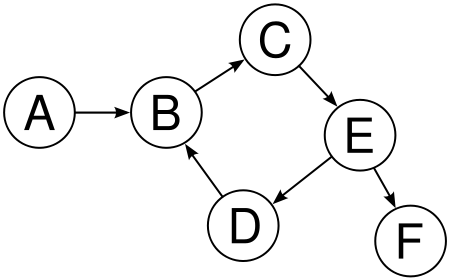## Topological Sorting (Only for Directed)

Topological sorting of a directed graph in graph theory is just a simple linear ordering of the nodes of the graph in such a way such that for every directed edge uv from vertex u to vertex v, u comes before v in the ordering.

Example-Here one of the topologically sorted sequence can be

A,B,C,E,D,F

# Algorithm to find the sequence-

To find a topologically sorted sequence, the following algorithm is used.

1. We start from any vertex and apply a DFS on the node and start searching for all its childs.

2. We do DFS on a certain node until all its child are visited (using

DFS method)

3. After all the children of a the node are visited, we push that into a stack.

4. Popping out the stack after visiting all nodes through this algorithm will give us the topologically sorted sequence.

# Code in C++

Some Practice Problems